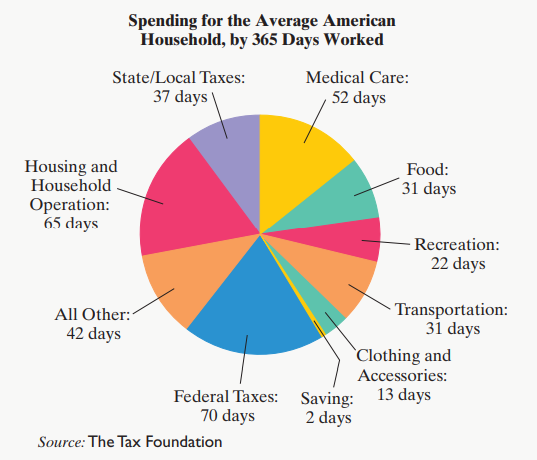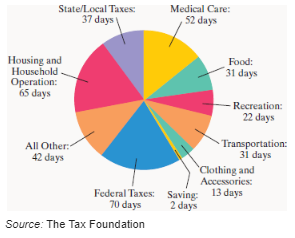Thinking Mathematically - 6 Edition - Chapter 8.1 - Problem 51
Register Now

Join StudySoup

Get Full Access to Thinking Mathematically - 6 Edition - Chapter 8.1 - Problem 519780321867322

# The circle graph shows a breakdown of spending for the

Thinking Mathematically | 6th Edition

Problem 51

The circle graph shows a breakdown of spending for the average U.S. household using 365 days worked as a basis of comparison. Use this information to solve Exercises 51 – 52. Round answers to the nearest tenth of a percent.What percentage of work time does the average U.S. household spend paying for federal taxes?

Accepted Solution
Step-by-Step Solution:

Problem 51

The circle graph shows a breakdown of spending for the average U.S. household using 365 days worked as a basis of comparison. Round answers to the nearest tenth of a percent.

Spending for the Average American Household, by 365 Days WorkedWhat percentage of work time does the average U.S. household spend paying for federal taxes?

Step by Step Solution

Step 1 of 2

Consider the graph from the textbook.

The objective is to find the percentage of work that was spent by the average U.S. household for federal taxes.

###### Chapter 8.1, Problem 51 is Solved

Step 2 of 2

Unlock Textbook Solution